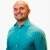# Learn how to graph an absolute value equation with a horizontalThis lesson will look at an example problem involving absolute value equations. This lesson will give an explanation of how to graph an absolute value equation with a horizontal with a step by step look to... This lesson will look at an example problem involving absolute value equations. This lesson will give an explanation of how to graph an absolute value equation with a horizontal with a step by step look to better explain the concept.
More... Collapse
2 Views